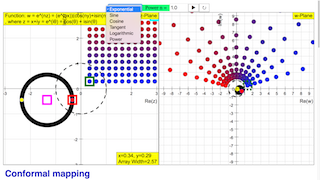Description

# Conformal mapping

### Simulation window

In the z-plane (left) there is a quadratic point array of predefined width and position. Its points are differentiated in color according to their imaginary value. The lower left edge point is accentuated in red;  it is connected to the origin by a green vector arrow. The array can be shifted in the plane, drawing the red point with the mouse. Its coordinates can be varied independently by two sliders x, y. Exact values can be written into the x,y number fields; they may be beyond the slider range. The array width can be changed by another slider; it can be contracted to a point.

In addition there is a circular color coded point array around the origin. Its center is highlighted in magenta. The two symmetric points at the real axis are accentuated in red and yellow. The circular array can be shifted with the mouse by drawing at its center. The diameter of the circular array can be varied by a radius slider and can also be contracted to a point.

In both planes there are black circles with radii characteristic for the special function (1, e , π). In the z-plane red lines define the limits of periodic regions or of mapping strips

The arrays of the z-plane are mapped into the w-plane by the complex function. The color coding is conserved in this process to identify specific rows of points. When the arrays are contracted to a point, one sees the mapping of this single point. Exact coordinates are shown when a point is marked with the mouse.

The play button starts an animation that shifts the quadratic array by a raster unit per second along a line that is interesting for the special function (real axis, imaginary axis, unit circle). When the array reaches the end of the scale, it jumps to the opposite limit. One can shift the array with the mouse, sliders or coordinate number fields while the animation is running. This way the plane can be quickly rastered. The pause button stops the animation.

The color coding is most distinct when the window is blown up to full screen size.

Functions

# The available functions provided in the Combobox, along with their equations, are as follows:

- Exponential:  w = e^(nz), where n is a real number

- Sine:  w = sin(z)

- Cosine: w = cos(z)

- Tangent:  w = tan(z)

- Logarithmic:  w = ln(z)

- Power:  w = z^n, where n is a real number

Authors

this file was created by Dieter Roess in July 2008

This simulation is part of

– Plus 2000 Examples from Physics”

ISBN 978-3-11-025005-3, Walter de Gruyter GmbH & Co. KG

### Translations

Code Language Translator Run### Software Requirements

SoftwareRequirements

 Android iOS Windows MacOS with best with Chrome Chrome Chrome Chrome support full-screen? Yes. Chrome/Opera No. Firefox/ Samsung Internet Not yet Yes Yes cannot work on some mobile browser that don't understand JavaScript such as..... cannot work on Internet Explorer 9 and below

### CreditsDieter Roess - WEH- Foundation; Tan Wei Chiong; Loo Kang Wee

[text]

### For Teachers

In the real numbers, we can express a relationship between two sets of numbers by mapping elements from one set to elements in another. We call this mapping a function.

To visualize this, we can plot out a graph of y against x, where y = f(x).

However, this is not possible with complex numbers, which are represented with a 2-dimensional plane. Thus, we map a complex number from one Argand plane to another Argand plane. By seeing how each function transforms a square array of points (red to blue) and the unit circle (black), we can reveal some very interesting properties of how complex numbers behave under a function.

The available functions are as follows:
- Exponential (w = e^z)
- Sine (w = sin(z))
- Cosine (w = cos(z))
- Tangent (w = tan(z))
- Logarithmic (w = ln(z))
- Power (w = z^n)

Research

[text]

[text]

### Version:

1. http://weelookang.blogspot.sg/2016/02/vector-addition-b-c-model-with.html improved version with joseph chua's inputs
2. http://weelookang.blogspot.sg/2014/10/vector-addition-model.html original simulation by lookang

[text]